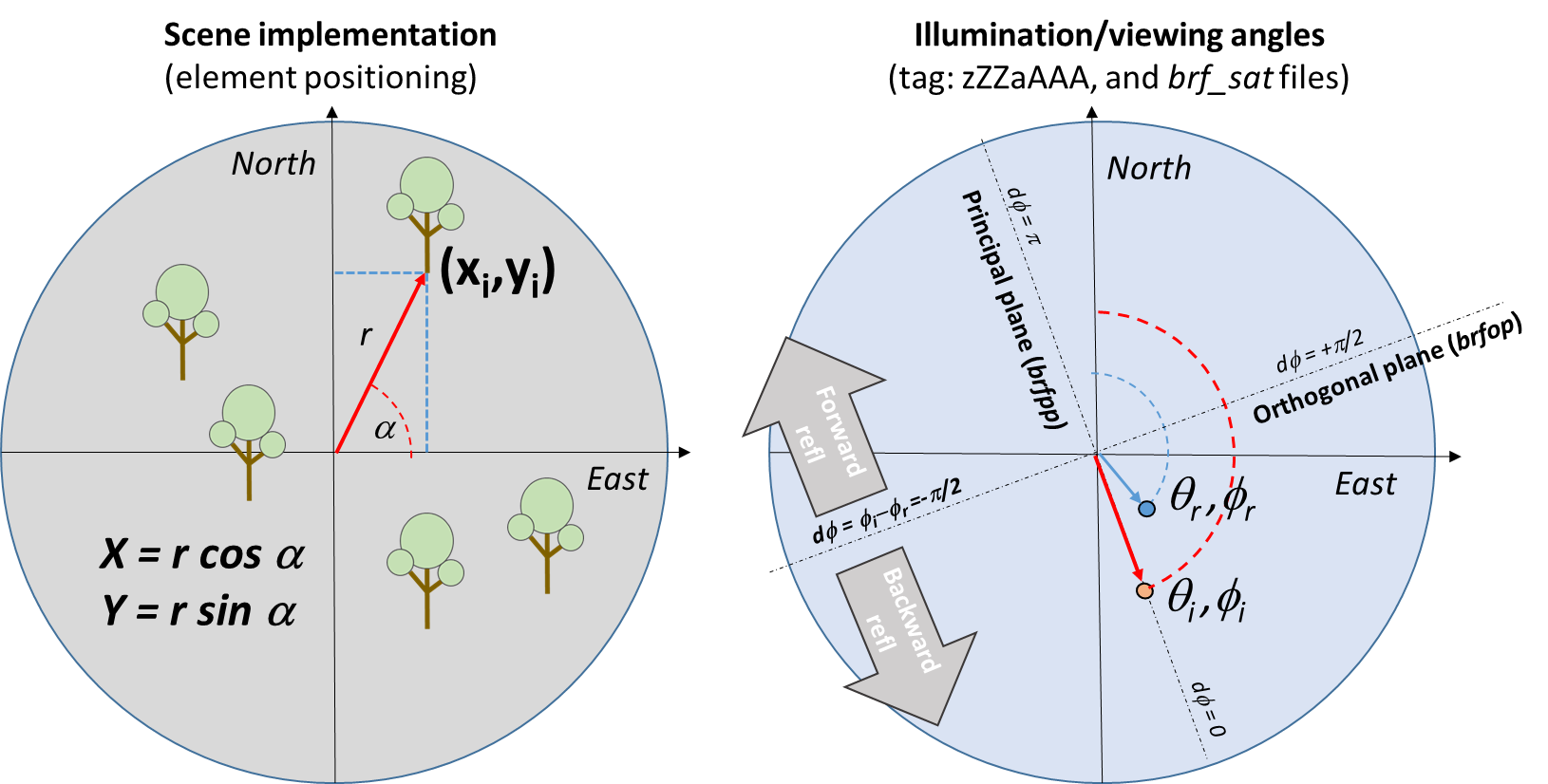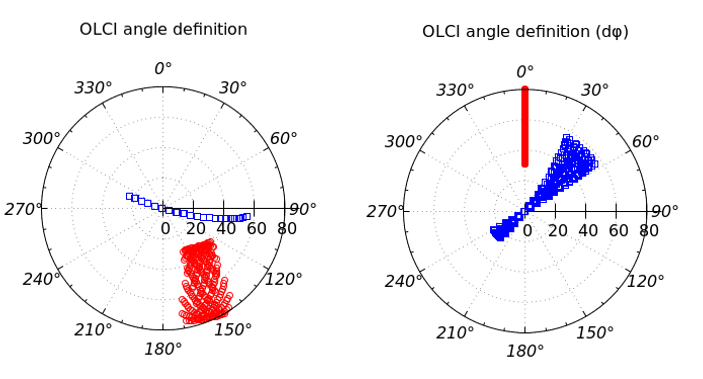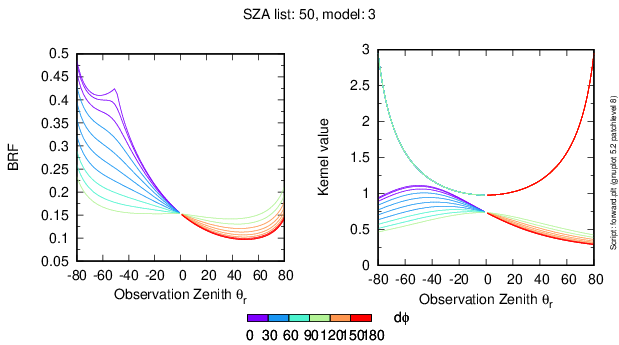RAMI website

# Definition

Guidance

This page contains valuable information regarding the generation of the various scene representations, as well as on the way the user simulated results are evaluated:

## Terminology

All remote sensing measurements acquired from space or airborne imaging sensors in the solar domain turn out to be strongly dependent on the particular geometry of illumination and observation at the time these measurements were made. A similar situation occurs in the spectral domain: different measurement values are obtained when a target is observed in different spectral bands. The reflectance of a geophysical medium (at a particular wavelength) is thus dependent on both the position of the Sun and the observer with respect to the target. Such a medium is called anisotropic, and the reflectance is characterized as bi-directional. By contrast, a perfectly reflecting, isotropic surface, i.e., a system that would reflect the incoming light equally in all directions, is called Lambertian.

The mathematical function describing the anisotropic reflectance is called Bidirectional Reflectance Distribution Function (BRDF). For practical reasons, the reflectance of a target measured in a specific direction is normalized by the reflectance of a reference panel, (ideally) a Lambertian surface, illuminated and observed under identical geometric conditions. The result of this normalisation defines the Bi-directional Reflectance Factor (BRF), which is related to BRDF by $BRF = \pi BRDF$.Definition of the polar angles adopted in RAMI-V. (1) The left hand side panel shows the convention adopted for the creation of the scenes, except differently specified. (2) The right hand side panel illustrates the convention adopted for the definition of illumination ($\theta_i$,$\phi_i$) and viewing ($\theta_r$,$\phi_r$) angles.

#### References

Nicodemus, F. E., J. C. Richmond, J. J. Hsia, I. W. Ginsberg, and T. Limperis, (1977) Geometrical Considerations and Nomenclature for Reflectance, US Department of Commerce, National Bureau of Standards, NBS Monograph No. 160, Washington, DC.
Martonchik, J. V., C. J. Bruegge, and A. Strahler (2000) A Review of Reflectance Nomenclature Used in Remote Sensing, Remote Sensing Reviews, 19, 9-20.
Verstraete, Michel M. and Bernard Pinty(2000) Environmental Information Extraction from Satellite Remote Sensing Data, in Geophysical Monograph No. 114, Edited by P. Kasibhatla, M. Heiman, P. Rayner, N. Mahowald, R. Prinn, Ronald and D. Hartley, American Geophysical Union, Washington, D.C., p. 125-137.
Schaepman-Strub, G., M.E. Schaepman, T.H. Painter, S. Dangel and J.V. Martonchik, (2006) Reflectance quantities in optical remote sensing - Definitions and case studies, Remote Sensing of Environment, 103 (1), 27-42.

## Sign convention for the zenith and azimuth angles

### Implementation of the scene

Direction cosines ($D_x$,$D_y$,$D_z$) are often used to define the spatial orientation of the primitives which define the foliage. They are given by the scalar product of the axes vectors $e_x$,$e_j$,$e_k$ with the vector which define the element orientation $\vec{v}$, normalized by the vector norm $\|{v}\|$.
Tree positions are given in files of ($x_c$,$y_c$,$z_c$) coordinates, or alternatively using polar coordinates (r,$\alpha$) as defined in the left hand side panel of Figure 1 (for $z_c$=contant).
For heterogeneous scenes graphical descriptions will be provided case by case to clarify these nomenclatures.

### Illumination and Observation angles

• Zenith ($\theta_i$) angles are counted from the local vertical and are always reported as a positive value [$0:\pi/2$].
• Azimuth angles ($\phi_i$) are arbitrarily counted from the positive y-axis (North) in clockwise (CW) direction, and are given as values in the range [$-\pi:+\pi$].
Note that RAMI-V adopts a different definition of the illumination and viewing angles with respect to the previous phases. This has been done to adopt the same convention used in Earth Observation remote sensing.
In RAMI 1 to RAMI 4, it was adopted a counter-clock-wise (CCW) positive variation of the azimuth angles, starting from East.
• As in the previous RAMI phases, the relative azimuth $\delta\phi$ is used as state variable in calculation and reports, and it is computed as $d\phi = \phi_i-\phi_r$, between the Sun and the observer. Then, it should be converted to the range ]$-\pi:\pi$]. A convenient way to perform the conversion of an azimuth angle to the above range is to use the $\phi' = \arctan(x,y) = \arctan(\cos(\phi),\sin(\phi))$.
• In case your RTM code requires the absolut value of viewing azimuth angles ($\phi_r$), the above formula should be inverted to obtain it: $\phi_r=\phi_i-d\phi$.

• The forward reflectance conditions are characterize by $|d\phi|>\pi/2$.
The backward reflectance conditions are characterize by $|d\phi|<\pi/2$.
The principal plane of reflection is characterized by $d\phi = 0$ (backward, hot-spot), or $d\phi=\pi$ (forward, sun-glint).
And the orthogonal plane of reflection by $d\phi=\pm\pi/2$.Definition of the polar angles adopted in RAMI-V. Polar plot of illumination (red) and observation (blue) angles observed over a particular target, and with OLCI and RAMI-V definitions. The azimuth difference in the second line are defined as $d\phi=\phi_i-\phi_r$.

## Leaf angle distribution functions

The spatial orientation of a leaf is described by the direction of its normal $\Omega_L(\theta_L,\phi_L)$ to the upper surface, where $\theta_L$ is the inclination angle of the leaf normal, and $\phi_L$ is the azimuthal angle of the outward normal. Consider a horizontally homogeneous leaf layer of unit thickness at height $z$, and let $\hat{g}_L(z,\Omega_L$) representing the sum of areas of all leaves (or parts thereof) whose normals fall within an incremental solid angle around the direction $\Omega_L$.
Here all leaves are assumed to face upward, so that all leaf normals are confined to the upper hemisphere ($2\pi+$), and $s^*L(z)$ is the total one sided area of all leaves in this horizontal layer. The leaf-normal distribution (LND) function $g_L(z,\Omega_L)= \hat{g}_L(z,\Omega_L)/{s^*L(z)}$, denotes the fraction of total leaf area in the horizontal layer of unit thickness at height z whose normals fall within unit solid angle around the direction $\Omega_L$, and must satisfy the following normalization criterion: $$\frac{1}{2\pi}\int_{2\pi+}g_L(\Omega_L)d\Omega_L=\frac{1}{2\pi}\int_0^{2\pi}d\phi_L \int_0^{\pi/2}g_L(\theta_L,\phi_L)\sin\theta_L d\theta_L = 1$$ A variety of leaf angle distribution functions have been published in the literature. Within RAMI (and RAMI4PILPS), however, azimuthal invariance of the leaf normal distribution is assumed dependent only on $\theta_L$: $$g_L(\Omega_L) = g_L(\theta_L)$$ so that, $$\int_0^{\pi/2} g_L(\theta_L)\sin\theta_Ld\theta_L = 1$$
For the purpose of standardization and comparison the following formulae for $g^*(\theta_L)=g(\theta_L) \sin\theta_L$ are recommended:

#### Distributions from Bunnik (1978):

• Planophile:    $g^*(\theta_L) = \frac{2}{\pi}(1+\cos 2\theta_L)$
• Erectophile:    $g^*(\theta_L) = \frac{2}{\pi}(1-\cos 2\theta_L)$
• Uniform:    $g^*(\theta_L) = \frac{2}{\pi}$

#### Distributions from Goel and Strebel (1984):

$$g^*_{GS}= \frac{2}{\pi} \frac{\Gamma(\mu+\nu)}{\Gamma(\mu)\Gamma(\nu)} \bigg(1-\frac{2\theta_L}{\pi}\bigg)^{\mu-1} \bigg(\frac{2\theta_L}{\pi}\bigg)^{\nu-1}$$

where $\Gamma$ is the gamma function. The following parameters define the different distributions:

• Planophile: $\mu$ = 2.531 and $\nu$ = 1.096
• Erectophile: $\mu$ = 1.096 and $\nu$ = 2.531

The correlation between the distribution functions of Bunnik and those of Goel and Strebel are given by the latter to be 0.9989 for both the planophile and erectophile cases.

#### References

Bunnik , N. J. J., (1978) The Multispectral Reflectance of Shortwave Radiation of Agricultural Crops in Relation With Their Morphological and Optical Properties, in Mededelingen Landbouwhogeschool, Wageningen, The Netherlands, 175 pages.
Goel, Narendra S. and Strebel, D. E., (1984) Simple Beta Distribution Representation of Leaf Orientation in Vegetation Canopies, Agronomy Journal, 76, 800-803.

## The parametric RPV (Rahman-Pinty-Verstraete) model

The RPV model is a parametric model that allows to adequately represent surface anisotropy patterns. Through its mathematical formulation the RPV model splits a BRF field into an amplitude component ($\rho_0$) and an associated angular field describing the anisotropic behaviour of the surface under investigation. The latter is represented by the product of three separate functions accounting for both the illumination and viewing directions. Thus, overall, the RPV model formulates the BRF (or HDRF) of a surface as $$\rho(z_0,\mu_i,\mu_r,d\phi;\rho_0,\Theta,k,\rho_c) = \rho_0 \cdot M(k) \cdot F_{HG} (\Theta) \cdot H(\rho_c) = \\$$ $$= \rho_0 \frac{(\mu_i \mu_r)^{k-1}}{(\mu_i+\mu_r)^{1-k}} \frac{1-\Theta^2}{(1 +\Theta^2 + 2\Theta \cos g)^{1.5}} \bigg( 1+\frac{1-\rho_c}{1+G} \bigg)$$ where, $\mu=cos\theta$,
$\cos g=\cos\theta_i\cos\theta_r+\sin\theta_i\sin\theta_r\cos d\phi$, and
$G = (\tan^2\theta_i+\tan^2\theta_r-2\tan\theta_i\tan\theta_r\cos d\phi)^{0.5}$

Notice that in the $F_{HG}$ function, the term $-2\Theta \cos(\pi-g)$ as appers in Rahman et al.(1993), is replaced here by $+2\Theta \cos g$.

Symbols $\theta_r$ and $\theta_i$ represents the observation and illumination zenith angles, respectively. In the above formulation the relative azimuth angle $d\phi$ is zero when the source of illumination is behind the sensor.
The modified Minnaert angular function $M(k)$, set the overall shape of the angular field through the parameter $k$. Specifically, $k$ is close to 1.0 for a quasi-Lambertian surface (very limited angular variations of the spectral BRF field), for $k<1$ a bowl-shaped pattern dominates (the spectral BRF values increase with the view zenith angle in the orthogonal plane), and for $k>1$ a bell-shaped pattern dominates (i.e., the spectral BRF values decrease with the view zenith angle in the orthogonal plane).
The other angular functions serve to add more complexity/flexibility to the anisotropy classes described above; they allow for the accounting of asymmetrical shapes due to the possible imbalance between the backward and forward scattering regions, as well as, for the backscatter enhancement due to the hot spot effect.
The Henyey-Greenstein function $F_{HG}(\Theta)$ is used to establishes the degree of forward ($\Theta>0$) or backward ($\Theta<0$) scattering, depending on the sign of the parameter $\Theta$.
Finally, the $H(\rho_c)$ function allows to model the hotspot with its parameter $\rho_c$. In some case, $\rho_c$ is assumed to be equal to $\rho_0$.

For your convenience, a FORTRAN and a Gnuplot implementation of the RPV algorithm are packed into a zipped archive, which can be downloaded here:

rpv2.zip
English
(10.7 KB - ZIP archive)

On Linux these files can be compiled using a fortran compiler like gfortran, f95, etc. A statement like:

gfortran main2.f rpv2.f -o rpv2

whill thus generate an executable called rpv2. Next by typing:

./rpv2 > rpv-out.dat.new

the rpv binary will read the information stored in the file rpv-in.dat and then generate an output file called rpv-out.dat.new. The content of this file should be identical that of the rpv-out.dat file provided above.

To ensure that the RPV model is encoded properly, the following table provides graphical evidence as to the output of the RPV model for a variety of input parameter combinations. The corresponding input/output files are available rpv2-benchmark:2-D Plot representation of the BRF as generated by the RPV function for $\rho_0=0.075, \Theta=-0.25, k=0.55$, and $\theta_s=50^\circ$, for $d\phi$ increasing in steps of $10^\circ$ (left). The right hand side panel shows the Minneart (bowl-shaped, for $k=0.55$), and $F_{HG}$ kernels for the same viewing configurations. Plotting NOTE: in such a kind of plots, it is common to indicate with $\theta_r\le0$ the backward reflection.

#### References

The following references describe the RPV model in detail or provide additional relevant information on to the use of this model:

Rahman, H., M. M. Verstraete, and B. Pinty, (1993) Coupled surface-atmosphere reflectance (CSAR) model. 1. Model description and inversion on synthetic data, Journal of Geophysical Research, 98, 20,779-20,789.
Rahman, H., B. Pinty, and M. M. Verstraete, (1993) Coupled surface-atmosphere reflectance (CSAR) model. 2. Semi-empirical surface model usable with NOAA Advanced Very High Resolution Radiometer data, Journal of Geophysical Research, 98, 20,791-20,801.
Engelsen, O., B. Pinty, M. M. Verstraete, and J. V. Martonchik, (1996) Parametric Bidirectional Reflectance Factor Models: Evaluation, Improvements and Applications, EC Joint Research Centre, Technical Report No. EUR 16426 EN, 114 p.

## The parametric Ross-Li reciprocal model

The Ross-Li Reciprocal BRDF model (RLI), adopted by the MODIS/Albedo-BRDF team [Strahler et al, 1999] represents the bidirectional reflectance features through the sum of three kernel functions ($K_i$) and corresponding parameters ($f_i$) as

The various kernels look as follows

where

\begin{equation*} O = \frac{1}{\pi} (t- \sin t \cos t )(\sec\theta_i' + \sec\theta_r') \end{equation*} \begin{equation*} \cos t = \frac{h}{b} \frac{\sqrt{D^2+(\tan\theta_i'\tan\theta_r'\sin d\phi)^2}}{\sec\theta_i' + \sec\theta_r'}, \frac{h}{b} = 2 \end{equation*} \begin{equation*} D = \sqrt{\tan^2\theta_i' + \tan^2\theta_r' -2 \tan\theta_i'\tan\theta_r'\cos d\phi} \end{equation*} \begin{equation*} cos\xi' = \cos\theta_i' \cos\theta_r' + \sin\theta_i' \sin\theta_r'\cos d\phi \end{equation*} \begin{equation*} \theta' = \tan^{-1}\left(\frac{b}{r} \tan\theta\right), \frac{b}{r} = 1 \end{equation*}

$K_{vol}$ and $K_{geo}$ are known also as Ross-Thick and Li-Sparse kernels, and represent respectively the portion of reflectance related to the volume of the canopy (body reflectance) and the geometric effects of mutual shadowing between the different trees.

#### Reference

A. H. Strahler, J.-P. Muller, MODIS Science Team Members, (1999) MODIS BRDF/Albedo Product: Algorithm Theoretical Basis Document Version 5.0, URL: https://modis.gsfc.nasa.gov/data/atbd/atbd_mod09.pdf

## RT model technicalities

If applicable to the participating RT model, cyclic boundary conditions must be applied to all test cases unless specified otherwise on the individual measurement description pages. In the case of scenes with topography, this implies a repetition of the topographic features at scales equal to the scene dimension.

By default RT simulations are carried out with respect to a reference plane. Only those portions of the incoming and exiting radiation that pass through this reference plane are to be considered in the various measurements. Unless specified otherwise, the default reference plane covers the entire test case area (known as the "scene") and is located at the top-of-the-canopy height, that is, just above the highest structural element in the scene. The spatial extend of the reference plane can be envisaged as the (idealised) boundaries of the IFOV of a perfect sensor looking at a 'flat' surface located at the height level of the reference plane.

## Model Proficiency Assessments

#### Local angular model deviation, $\delta_m(\theta_v)$

A criterion to quantify inter-model variability is the distance between BRF fields generated under identical geophysical and geometrical conditions. Specifically the local angular deviation, is computed to estimate how a given model $m$, behaves with respect to an ensemble of other models, at the specific exiting angle ($\theta_v$) for an ensemble of $N_{\theta_0}$ illumination conditions, the structural scenario $s$ (out of a total of $N_s$ scenes test cases), and the wavelength $\lambda$ (out of a total of $N_λ$ wavelengths).
The deviation is then normalized over all simulations $N = N_{\theta_0} + N_s + N_\lambda + N_m$:

$$\delta_m(\theta_v) = \frac{1}{N} \sum_{i=1}^{N_{\theta_0}} \sum_{s=1}^{N_s} \sum_{\lambda=1}^{N_\lambda} \sum_{k=1,k \ne m}^{N_m} \frac{|\rho_m(\theta_v,i,s,\lambda)-\rho_k(\theta_v,i,s,\lambda)|} {\rho_m(\theta_v,i,s,\lambda)+\rho_k(\theta_v,i,s,\lambda)}$$

Similar metrics can be designed to examine the model discrepancies for each geometrical condition of illumination and/or observation, scene and wavelength. They can all be derived following the generic form of the above equation.
For instance the appropriate metrics for analyzing the model discrepancies separately for each wavelength would be:

$$\delta_m(\theta_r,\lambda) = \frac{1}{N'} \sum_{i=1}^{N_{\theta_0}} \sum_{s=1}^{N_s} \sum_{k=1,k \ne m}^{N_m} \frac{|\rho_m(\theta_r,i,s,\lambda)-\rho_k(\theta_r,i,s,\lambda)|} {\rho_m(\theta_r,i,s,\lambda)+\rho_k(\theta_r,i,s,\lambda)}$$

#### Global angular model deviations, $\delta_m$

An estimate of the global angular model deviation can be obtained by integrating over all available viewing conditions of the local angular model deviation:

$$\delta_m = \frac{1}{N_{\theta_r}} \sum_{i=1}^{N_{\theta_r}}\delta_m(\theta_r)$$